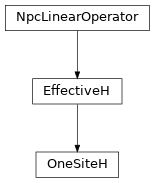# OneSiteH¶

Inheritance DiagramMethods

 OneSiteH.__init__(env, i0[, combine, move_right]) Return the hermitian conjugate of self. Combine LP and RP with W to form LHeff and RHeff, depending on the direction. Combine the legs of theta, such that it fits to how we combined the legs of self. OneSiteH.matvec(theta) Apply the effective Hamiltonian to theta. Contract self to a matrix. OneSiteH.update_LP(env, i[, U]) Equivalent to env.get_LP(i, store=True); optimized for combine. OneSiteH.update_RP(env, i[, VH]) Equivalent to env.get_RP(i, store=True); optimized for combine.

Class Attributes and Properties

class tenpy.algorithms.mps_common.OneSiteH(env, i0, combine=False, move_right=True)[source]

Bases: EffectiveH

Class defining the one-site effective Hamiltonian for Lanczos.

The effective one-site Hamiltonian looks like this:

|        .---    ---.
|        |    |     |
|       LP----W0----RP
|        |    |     |
|        .---    ---.


If combine is True, we define either LHeff as contraction of LP with W (in the case move_right is True) or RHeff as contraction of RP and W.

Parameters
• env (MPOEnvironment) – Environment for contraction <psi|H|psi>.

• i0 (int) – Index of the active site if length=1, or of the left-most active site if length>1.

• combine (bool) – Whether to combine legs into pipes. This combines the virtual and physical leg for the left site (when moving right) or right side (when moving left) into pipes. This reduces the overhead of calculating charge combinations in the contractions, but one matvec() is formally more expensive, $$O(2 d^3 \chi^3 D)$$. Is originally from the wo-site method; unclear if it works well for 1 site.

• move_right (bool) – Whether the the sweep is moving right or left for the next update.

length

Number of (MPS) sites the effective hamiltonian covers.

Type

int

acts_on

Labels of the state on which self acts. NB: class attribute. Overwritten by normal attribute, if combine.

Type

list of str

combine, move_right

See above.

Type

bool

LHeff, RHeff

Only set if combine, and only one of them depending on move_right. If move_right was True, LHeff is set with labels '(vR*.p0)', 'wR', '(vR.p0*)' for bra, MPO, ket; otherwise RHeff is set with labels '(p0*.vL)', 'wL', '(p0, vL*)'

Type

Array

LP, W0, RP

Tensors making up the network of self.

Type

Array

matvec(theta)[source]

Apply the effective Hamiltonian to theta.

Parameters

theta (Array) – Labels: vL, p0, vR if combine=False, (vL.p0), vR or vL, (p0.vR) if True (depending on the direction of movement)

Returns

Product of theta and the effective Hamiltonian.

Return type

theta Array

combine_Heff(env)[source]

Combine LP and RP with W to form LHeff and RHeff, depending on the direction.

In a move to the right, we need LHeff. In a move to the left, we need RHeff. Both contain the same W.

Parameters

env (MPOEnvironment) – Environment for contraction <psi|H|psi>.

combine_theta(theta)[source]

Combine the legs of theta, such that it fits to how we combined the legs of self.

Parameters

theta (Array) – Wave function with labels 'vL', 'p0', 'vR'

Returns

theta – Wave function with labels '(vL.p0)', 'vR'

Return type

Array

to_matrix()[source]

Contract self to a matrix.

Return the hermitian conjugate of self.

update_LP(env, i, U=None)[source]

Equivalent to env.get_LP(i, store=True); optimized for combine.

Parameters
• env (MPOEnvironment) – The same environment as given during class initialization.

• i (int) – We update the part left of site i. Can optimize if i == i0 and combine is True.

• U (None | Array) – The tensor on the left-most site self acts on, with combined legs after SVD. Only used if trying to optimize.

update_RP(env, i, VH=None)[source]

Equivalent to env.get_RP(i, store=True); optimized for combine.

Parameters
• env (MPOEnvironment) – The same environment as given during class initialization.

• i (int) – We update the part right of site i. Can optimize if i == i0 + 2 - length and combine is True.

• U (None | Array) – The tensor on the right-most site self acts on, with combined legs after SVD. Only used if trying to optimize.